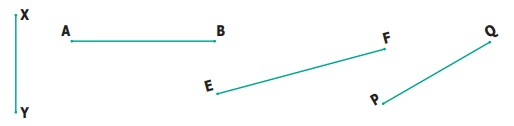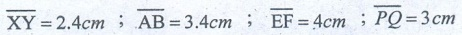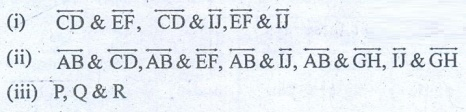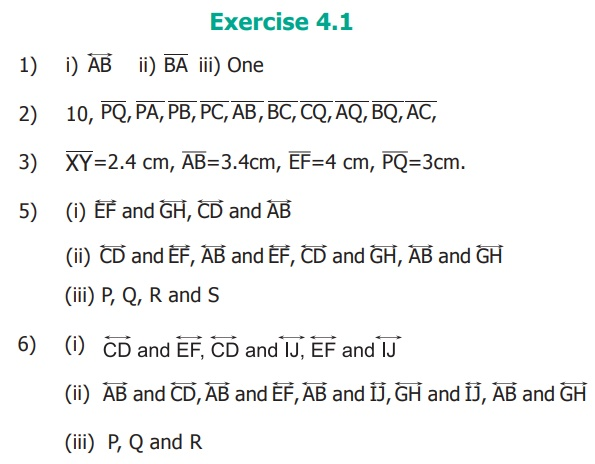Home | | Maths 6th Std | Exercise 4.1

# Exercise 4.1

6th Maths : Term 1 Unit 4 : Geometry | Text Book Back Exercises Questions with Answers, Solution

Exercise 4.1

1. Fill in the blanks.

(i) A line through two end points ‘A’ and ‘B’ is denoted by ____________. Answer:(ii) A line segment from point ‘B’ to point ‘A’ is denoted by ____________. Answer:(iii) A ray has ____________ end point(s). Answer: one

2. How many line segments are there in the given line? Name them.Answer: 10 line segments are there in the given line

They are3. Measure the following line segments.4. Construct a line segment using ruler and compass.5. From the given figure

(i) identify the parallel lines

(ii) identify the intersecting lines

(iii) name the points of intersection.6. From the given figure, name the

(i) parallel lines

(ii) intersecting lines

(iii) points of intersection.7. From the given figure, name

(i) all pairs of parallel lines.

(ii) all pairs of intersecting lines.

(iii) pair of lines whose point of intersection is ‘V’.

(iv) point of intersection of the lines ‘ l2 ’ and ‘l3’.

(v) point of intersection of the lines ‘l1’ and ‘l5’.(i) ‘l3’ & ‘l4’ , ‘l4’ & ‘l5’, ‘l3’  & ‘l5’

(ii) ‘l1’ & ‘l2’ , ‘l1’ & ‘l3’, ‘l1’  & ‘l4’ ‘l1’ & ‘l5’ , ‘l2’ & ‘l3’,

(iii) ‘l1’  & ‘l2’

(iv) Q

(v) U

Objective Type Questions

8. The number of line segments inis

(a) 1

(b) 2

(c) 3

(d) 4

9. A line is denoted asAnswer: (d)(iii) P, Q and R

7) (i) l3 and l4 , l4 and l5 , l3 and l5

(ii) l1 and l2, l1 and l3 , l1 and l4 , l1 and l5 , l2 and l3 , l2 and l4 , l2 and l5

(iii) l1 and l2

(iv) Q

(v) U

8) c) 3

9) c)Tags : Questions with Answers, Solution | Geometry | Term 1 Chapter 4 | 6th Maths , 6th Maths : Term 1 Unit 4 : Geometry
Study Material, Lecturing Notes, Assignment, Reference, Wiki description explanation, brief detail
6th Maths : Term 1 Unit 4 : Geometry : Exercise 4.1 | Questions with Answers, Solution | Geometry | Term 1 Chapter 4 | 6th Maths